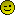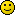Fabrication Everything From JBwelded/Fluxcored downpipes to Equal length SS Manifolds.

# Formula for making precise PIE CUT BENDS............!!!!

0.0 BAR

Join Date: May 2005
Posts: 0Formula for making precise PIE CUT BENDS............!!!!

To make precise pie cuts bends I have provided a nice simple formula.
This formula makes a bend that is whatever angle you desire.

CLR = Center Line Radius (radius of the bend measured through the center of the tubing)
D = Diameter of Tube _____
L = Length of long side of pie cut - the top of this pie cut -> \____/
Angle = angle to cut pie section which, this angle is measured perpendicular to the length of the tubing
Bend Angle = The angle of the bend
N = number of pie sections to complete bend
* means multiply

L = [ ( 2 * CLR ) + D ] * [ sin ( Angle ) ]

Angle = Bend Angle / ( 2 * N )

Example:

You want to make a bend that is 120 degrees, uses 3" diameter tubing with a CLR of 4", and 5 pie sections.

Angle = Bend Angle / ( 2 * N )
Angle = 120 / ( 2 * 5 )
Angle = 12

L = [ ( 2 * CLR ) + D ] * [ sin ( Angle ) ]
L = [ ( 2 * 4" ) + 3" ] * [ sin ( 12 ) ]
L = [ 8" + 3" ] * [ sin ( 12 ) ]
L = [ 11" ] * [ sin ( 12 ) ]
L = [ 11" ] * [ sin ( 12 ) ]
L = [ 11" ] * [ 0.208 ]
L = 2.288"

[size=16pt]L = 2.288"
Angle = 12 degrees
_____
Now you need to cut 5 of these -> \____/ with the top part measuring 2.288" in length.

Here is an Excel ------sheet that t_cel_t created that uses this formula for you mathematically challenged individuals. Pie Cut Excel Sheet

EDIT*

Here's the downpipe and mani I did for my car. It's 3", uses 5 pieces per 90*, and has a CLR of 3"
[/size]3.0 BAR

Join Date: Jun 2006
Posts: 3,256Re: Formula for making precise PIE CUT BENDS............!!!!

nice! This should come in handy for me very soon when I fab some cooler piping.0.0 BAR

Join Date: Mar 2004
Posts: 0Re: Formula for making precise PIE CUT BENDS............!!!!

My formula is eyeball x pipe / radius of the imaginary pipe in my mind= close enough.3.0 BAR

Join Date: Apr 2006
Posts: 3,015Re: Formula for making precise PIE CUT BENDS............!!!!

My formula is eyeball x pipe / radius of the imaginary pipe in my mind= close enough.
x2 if it looks like it will work ill maike it fit!!3.0 BAR

Join Date: Aug 2003
Location: C-dale, NEPA
Posts: 4,667Re: Formula for making precise PIE CUT BENDS............!!!!

My formula is eyeball x pipe / radius of the imaginary pipe in my mind= close enough.
Lol that's how I used to do it at first, then I smartened up. Framing square ftw, has all the angles on there. I just figure out how many bends I want to make the turn and find what angle it'll be for them with the square.0.0 BAR

Join Date: Dec 2003
Posts: 0Re: Formula for making precise PIE CUT BENDS............!!!!

My formula is eyeball x pipe / radius of the imaginary pipe in my mind= close enough.0.0 BAR

Join Date: May 2007
Posts: 30Re: Formula for making precise PIE CUT BENDS............!!!!

My formula is eyeball x pipe / radius of the imaginary pipe in my mind= close enough.
haha thats awsome1.0 BAR

Join Date: Jan 2006
Posts: 295Re: Formula for making precise PIE CUT BENDS............!!!!

i seen this on here before, but make it a sticky!!!!!!!!!!!!!!!!!!
thanks0.0 BAR

Join Date: May 2006
Posts: 23Re: Formula for making precise PIE CUT BENDS............!!!!

thank you0.0 BAR

Join Date: Nov 2006
Posts: 0Re: Formula for making precise PIE CUT BENDS............!!!!

Why would nobody care about this? It's a great bit of information, I say sticky in the Fabrication section.Show Printable VersionEmail this Page